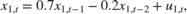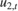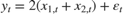# Filter Time-Varying Diffuse State-Space Model

This example shows how to generate data from a known model, fit a diffuse state-space model to the data, and then filter the states.

Suppose that a latent process comprises an AR(2) and an MA(1) model. There are 50 periods, and the MA(1) process drops out of the model for the final 25 periods. Consequently, the state equation for the first 25 periods isand for the last 25 periods, it iswhereandare Gaussian with mean 0 and standard deviation 1.

Assuming that the series starts at 1.5 and 1, respectively, generate a random series of 50 observations fromand.

T = 50; ARMdl = arima('AR',{0.7,-0.2},'Constant',0,'Variance',1); MAMdl = arima('MA',0.6,'Constant',0,'Variance',1); x0 = [1.5 1; 1.5 1]; rng(1); x = [simulate(ARMdl,T,'Y0',x0(:,1)),... [simulate(MAMdl,T/2,'Y0',x0(:,2));nan(T/2,1)]]; 

The last 25 values for the simulated MA(1) data are NaN values.

The latent processes are measured usingfor the first 25 periods, andfor the last 25 periods, whereis Gaussian with mean 0 and standard deviation 1.

Use the random latent state process (x) and the observation equation to generate observations.

y = 2*sum(x','omitnan')' + randn(T,1); 

Together, the latent process and observation equations make up a state-space model. The coefficients are unknown parameters, the state-space model isfor the first 25 periods,for period 26, andfor the last 24 periods.

Write a function that specifies how the parameters in params map to the state-space model matrices, the initial state values, and the type of state.

 % Copyright 2015 The MathWorks, Inc. function [A,B,C,D,Mean0,Cov0,StateType] = diffuseAR2MAParamMap(params,T) %diffuseAR2MAParamMap Time-variant diffuse state-space model parameter %mapping function % % This function maps the vector params to the state-space matrices (A, B, % C, and D) and the type of state (StateType). From periods 1 to T/2, the % state model is an AR(2) and an MA(1) model, and the observation model is % the sum of the two states. From periods T/2 + 1 to T, the state model is % just the AR(2) model. The AR(2) model is diffuse. A1 = {[params(1) params(2) 0 0; 1 0 0 0; 0 0 0 params(3); 0 0 0 0]}; B1 = {[1 0; 0 0; 0 1; 0 1]}; C1 = {params(4)*[1 0 1 0]}; Mean0 = []; Cov0 = []; StateType = [2 2 0 0]; A2 = {[params(1) params(2) 0 0; 1 0 0 0]}; B2 = {[1; 0]}; A3 = {[params(1) params(2); 1 0]}; B3 = {[1; 0]}; C3 = {params(5)*[1 0]}; A = [repmat(A1,T/2,1);A2;repmat(A3,(T-2)/2,1)]; B = [repmat(B1,T/2,1);B2;repmat(B3,(T-2)/2,1)]; C = [repmat(C1,T/2,1);repmat(C3,T/2,1)]; D = 1; end 

Save this code as a file named diffuseAR2MAParamMap on your MATLAB® path.

Create the diffuse state-space model by passing the function diffuseAR2MAParamMap as a function handle to dssm.

Mdl = dssm(@(params)diffuseAR2MAParamMap(params,T)); 

dssm implicitly creates the diffuse state-space model. Usually, you cannot verify diffuse state-space models that are implicitly created.

To estimate the parameters, pass the observed responses (y) to estimate. Specify an arbitrary set of positive initial values for the unknown parameters.

params0 = 0.1*ones(5,1); EstMdl = estimate(Mdl,y,params0); 
Method: Maximum likelihood (fminunc) Effective Sample size: 48 Logarithmic likelihood: -110.313 Akaike info criterion: 230.626 Bayesian info criterion: 240.186 | Coeff Std Err t Stat Prob --------------------------------------------------- c(1) | 0.44041 0.27687 1.59069 0.11168 c(2) | 0.03949 0.29585 0.13349 0.89380 c(3) | 0.78364 1.49222 0.52515 0.59948 c(4) | 1.64260 0.66736 2.46134 0.01384 c(5) | 1.90409 0.49374 3.85648 0.00012 | | Final State Std Dev t Stat Prob x(1) | -0.81932 0.46706 -1.75420 0.07940 x(2) | -0.29909 0.45939 -0.65107 0.51500 

EstMdl is a dssm model containing the estimated coefficients. Likelihood surfaces of state-space models might contain local maxima. Therefore, try several initial parameter values, or consider using refine.

Filter the states and obtain state forecasts by passing EstMdl and the observed responses to filter.

[~,~,Output]= filter(EstMdl,y); 

Output is a T-by-1 structure array that contains the filtered states and state forecasts.

Convert Output to a table.

OutputTbl = struct2table(Output); OutputTbl(1:10,1:5) % Display first ten rows of first five variables 
ans = 10x5 table LogLikelihood FilteredStates FilteredStatesCov ForecastedStates ForecastedStatesCov _____________ ______________ _________________ ________________ ___________________ {0x0 double} {0x0 double} {0x0 double} {0x0 double} {0x0 double} {0x0 double} {0x0 double} {0x0 double} {0x0 double} {0x0 double} {[ -2.3218]} {4x1 double} {4x4 double} {4x1 double} {4x4 double} {[ -2.4464]} {4x1 double} {4x4 double} {4x1 double} {4x4 double} {[ -3.8758]} {4x1 double} {4x4 double} {4x1 double} {4x4 double} {[ -2.5212]} {4x1 double} {4x4 double} {4x1 double} {4x4 double} {[ -1.9016]} {4x1 double} {4x4 double} {4x1 double} {4x4 double} {[ -1.9284]} {4x1 double} {4x4 double} {4x1 double} {4x4 double} {[ -2.4110]} {4x1 double} {4x4 double} {4x1 double} {4x4 double} {[ -2.6502]} {4x1 double} {4x4 double} {4x1 double} {4x4 double} 

The first two rows of the table contain empty cells or zeros, which correspond to the observations required to initialize the diffuse Kalman filter. That is, SwitchTime is 2.

SwitchTime = 2; 

Extract the filtered and forecasted states from the table. Recall that the two different states are in positions 1 and 3. The states in positions 2 and 4 help to specify the processes of interest.

stateIdx = [1 3]; % State indices of interest FilteredStates = NaN(T,numel(stateIdx)); ForecastedStates = NaN(T,numel(stateIdx)); for t = (SwitchTime + 1):T maxInd = size(Output(t).FilteredStates,1); mask = stateIdx <= maxInd; FilteredStates(t,mask) = Output(t).FilteredStates(stateIdx(mask),1); ForecastedStates(t,mask) = Output(t).ForecastedStates(stateIdx(mask),1); end FilteredStates(1:SwitchTime,:) = 0; ForecastedStates(1:SwitchTime,:) = 0; 

Plot the true state values, the filtered states, and the state forecasts together for each model.

figure plot(1:T,x(:,1),'-k',1:T,FilteredStates(:,1),':r',... 1:T,ForecastedStates(:,1),'--g','LineWidth',2); title('AR(2) State Values') xlabel('Period') ylabel('State Value') legend({'True state values','Filtered state values','State forecasts'}); figure plot(1:T,x(:,2),'-k',1:T,FilteredStates(:,2),':r',... 1:T,ForecastedStates(:,2),'--g','LineWidth',2); title('MA(1) State Values') xlabel('Period') ylabel('State Value') legend({'True state values','Filtered state values','State forecasts'});